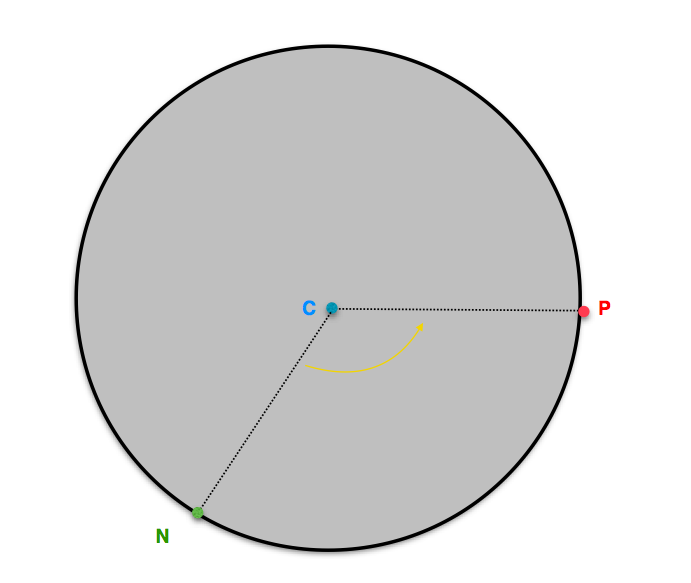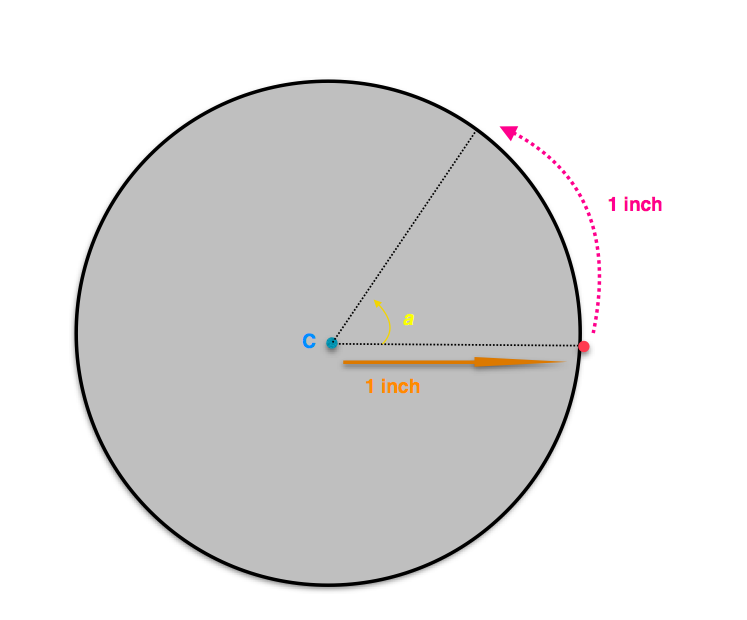Ferris Wheel
starstarstarstarstarstarstarstarstarstar
by Hannah Ryan
| 9 Questions
The toy ferris wheel has a radius of 13 inches pictured below:The point C is the center of the wheel and a point P on the circle is chosen. After the ferris wheel has moved forward a distance of d, the point P moves to a new point on the circle, marked N.A. Complete the table below, showing how the angle NCP varies with the distance d that the wheel has traveled forward:

Angle Measurements in Degrees:1
1
1 inch =
2
1
10 inches =
3
1
3 feet =
4
1
7 feet =
B. Remember that one radian is the measure of the angle cut out by a one unit length arc on a circle with radius one unit so m(a) = 1 radian in the picture below:More generally, a counterclockwise angle of r radians is the angle cut out by an arc of length r units on a unit circle.  Using this definition of radian angle measure, complete the table below, showing how the angle NCP varies with the distance d that the wheel has traveled forward:5
1
1 inch =
6
1
10 inches =
7
1
3 feet =
8
1
7 feet =
9
1
C. What is the relationship between the measure of an angle in degrees and its measure in radians?